## How to Calculate and Solve for Vasquez-Beggs Gas Solubility in a Fluid | The Calculator Encyclopedia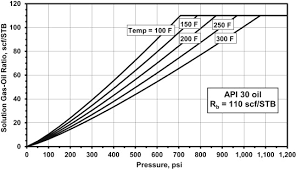The image above represents the Vasquez-Beggs Gas Solubility.

To compute for the Vasquez-Beggs gas solubility, five essential parameters are needed and these parameters are API Gravity (°API), Temperature (°Rankine) (T), Gas Gravity at Reference Separator Pressure (γgs), Gas Gravity at Actual Separator Psep and Tsep g) and Gas Solubility Parameter (C1, C2, C3).

The formula for calculating Vasquez-Beggs gas solubility:

Rs = C1 γgs γgC2 exp[C3(°API / T)]

Where:

Rs = Vasquez-Beggs Gas Solubitity
°API = API Gravity
T = Temperature (°Rankine)
γgs = Gas Gravity at Reference Separator Pressure
γg = Gas Gravity at Actual Separator Psep and Tsep
C1, C2, C3 = Gas Solubility Parameter

Let’s solve an example;
Find the Vasquez-Beggs gas solubility when the API Gravity is 24, Temperature is 180, gas gravity at reference separator pressure is 60, gas gravity at actual separator is 56, gas solubility parameter is 72, 66 and 45.

This implies that;

°API = API Gravity = 24
T = Temperature (°Rankine) = 180
γgs = Gas Gravity at Reference Separator Pressure = 60
γg = Gas Gravity at Actual Separator Psep and Tsep = 56
C1, C2, C3 = Gas Solubility Parameter = 72, 66, 45

Rs = C1 γgs γgC2 exp[C3(°API/ T)]
Rs = 72 x 60 x 5666 exp[45(24/ 180)]
Rs = 72 x 60 x 5666 exp[45(0.133)]
Rs = 72 x 60 x 5666 exp
Rs = 72 x 60 x 2.401e+115 exp
Rs = 72 x 60 x 2.401e+115 x 403.428
Rs = 6.974e+119

Therefore, the Vasquez-Beggs gas solubility is 6.974e+119.

## How to Calculate and Solve for Standing Gas Solubility Parameter | Nickzom Calculator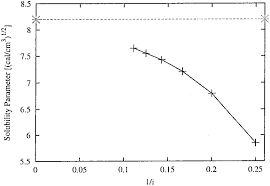The image above represents the standing gas solubility parameter.

To compute for the standing gas solubility parameter, two essential parameters are needed and these parameters are API Gravity (°API) and Temperature (°Rankine) (T).

The formula for calculating the standing gas solubility parameter:

x = 0.0125°API – 0.00091(T – 460)

Where:

x = Standing Gas Solubility Parameter
°API = API Gravity
T = Temperature (°Rankine)

Let’s solve an example;
Given that the API Gravity is 22 and the temperature is 120.
Find the standing gas solubility parameter?

This implies that;

°API = API Gravity = 22
T = Temperature (°Rankine) = 120

x = 0.0125°API – 0.00091(T – 460)
x = 0.0125(22) – 0.00091(120 – 460)
x = 0.275 – 0.00091(-340)
x = 0.275 – -0.3094
x = 0.5844

Therefore, the standing gas solubility parameter is 0.5844.

## How to Calculate and Solve for Standing Gas Solubility of a Fluid | The Calculator Encyclopedia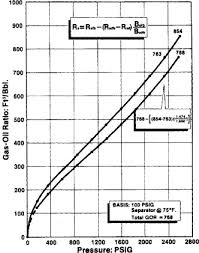The image above represents standing gas solubility.

To compute for the standing gas solubility, three essential parameters are needed and these are Solution Gas Specific Density (γg), System Pressure (P) and Standing Gas Solubility Parameter (x).

The formula for calculating the standing gas solubility:

Standing Rs = γg[(P / 18.2 + 1.4) 10x ]1.2048

Where:

Standing Rs = Standing Gas Solubility
γg = Solution Gas Specific Density
P = System Pressure
x = Standing Gas Solubility Parameter

Let’s solve an example;
Find the standing gas solubility when the solution gas specific density is 12, the system pressure is 40 and the standing gas solubility parameter is 20.

This implies that;

γg = Solution Gas Specific Density = 12
P = System Pressure = 40
x = Standing Gas Solubility Parameter = 20

Standing Rs = γg[(P / 18.2 + 1.4) 10x ]1.2048
Standing Rs = 12[(40 / 18.2 + 1.4) 1020 ]1.2048
Standing Rs = 12[(3.597802197802198) 1020]1.2048
Standing Rs = 12[(3.597802197802198) (100000000000000000000)]1.2048
Standing Rs = 121.2048
Standing Rs = 12[5.833292021408776e+24]
Standing Rs = 6.999950425690531e+25

Therefore, the standing gas solubility is 6.999950425690531e+25.

## How to Solve and Calculate for a Dimensionless Pressure Drop, Oil Rate, Oil FVF, Oil Viscosity in Well Testing | The Calculator Encyclopedia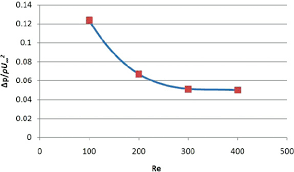The image above represents dimensionless pressure drop.

To compute for the dimensionless pressure drop, five essential parameters are needed and these parameters are Permeability-Thickness Product (kh), Pressure Drop (ΔP), Oil Rate (Qo), Oil Viscosity (μo) and Oil FVF (Bo).

The formula for calculating dimensionless pressure drop:

PD = khΔP / 141.2QoBoμo

Where;

PD = Dimensionless Pressure Drop
kH = Permeability-Thickness Product
ΔP = Pressure Drop
Qo = Oil Rate
μo = Oil Viscosity
Bo = Oil FVF

Let’s solve an example;
Find the dimensionless pressure drop with a permeability-thickness product of 70, a pressure drop of 30, an oil rate of 49, an oil viscosity of 19 and oil FVF of 31.

This implies that;

kh = Permeability-Thickness Product = 20
ΔP = Pressure Drop = 30
Qo = Oil Rate = 49
μo = Oil Viscosity = 19
Bo = Oil FVF = 31

PD = khΔP / 141.2QoBoμo
PD = 20 x 30/141.2 x 49 x 31 x 19
PD = 20 x 30/4075173.19
PD = 600/4075173.19
PD = 0.000147

Therefore, the dimensionless pressure drop is 0.000147 psi.

Calculating the Permeability-Thickness Product when Dimensionless Pressure Drop, Pressure Drop, Oil Rate, Oil Viscosity and Oil FVF.

kh = PD x 141.2QoBoμo / ΔP

Where;

kh = Permeability-Thickness Product
PD = Dimensionless Pressure Drop
ΔP = Pressure Drop
Qo = Oil Rate
μo = Oil Viscosity
Bo = Oil FVF

Let’s solve an example;
Find the permeability-thickness product with a dimensionless pressure drop of 28, a pressure drop of 16, an oil rate of 21, an oil viscosity of 18 and oil FVF of 24 .

This implies that;

PD = Dimensionless Pressure Drop = 28
ΔP = Pressure Drop =16
Qo = Oil Rate = 21
μo = Oil Viscosity = 18
Bo = Oil FVF = 24

kh = PD x 141.2QoBoμo / ΔP
kh = 28 x 141.2 x 21 x 24 x 18 / 16
kh = 35867059.8 / 16
kh = 2241691.2

Therefore, the permeability-thickness product is 2241691.2.

Calculating the Pressure Drop when Dimensionless Pressure Drop, Permeability-Thickness Product, Oil Rate, Oil Viscosity and Oil FVF.

ΔP = PD x 141.2QoBoμo / kh

Where;

ΔP = Pressure Drop
PD = Dimensionless Pressure Drop
kh = Permeability-Thickness Product
Qo = Oil Rate
μo = Oil Viscosity
Bo = Oil FVF

Let’s solve an example;
Find the pressure drop with a dimensionless pressure drop of 38, a permeability-thickness product of 24, an oil rate of 11, an oil viscosity of 8 and oil FVF of 12.

This implies that;

PD = Dimensionless Pressure Drop = 38
kh = Permeability-Thickness Product = 24
Qo = Oil Rate = 11
μo = Oil Viscosity = 8
Bo = Oil FVF = 12

ΔP = PD x 141.2QoBoμo / kh
ΔP = 38 x 141.2 x 11 x 12 x 8 / 24
ΔP = 5666073.6 / 24
ΔP = 236086.4

Therefore, the pressure drop is 236086.4.

## How to Calculate and Solve for Semi-Log Pressure-Time Slope, Permeability-Thickness Product, Oil Rate, Oil FVF, Oil Viscosity in Well Testing | Nickzom Calculator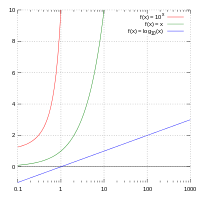The image above represents semi-log pressure-time slope.

To compute for the semi-log pressure-time slope, four essential parameters are needed and these parameters are Permeability-Thickness Product (kh), Oil Rate (Qo), Oil Viscosity (μo) and Oil FVF (Bo).

The formula for calculating the semi-log pressure-time slope:

m = 162.6QoBoμo / kh

Where;

m = Semi-Log Pressure-Time Slope
kh = Permeability-Thickness Product
Qo = Oil Rate
μo = Oil Viscosity
Bo = Oil FVF

Let’s solve an example;
Find the semi-log pressure-time slope, when permeability-thickness product is 12, oil rate is 17, oil viscosity is 21 and oil FVF is 13.7.

This implies that;

kh = Permeability-Thickness Product = 12
Qo = Oil Rate = 17
μo = Oil Viscosity = 21
Bo = Oil FVF = 13.7

m = 162.6QoBoμo / kh
m = 162.6 x 17 x 13.7 x 21 / 12
m = 795260.34 / 12
m = 66271.69

Therefore, the semi-log pressure-time slope is 66271.69.

Calculating the Permeability-Thickness Product when Semi-Log Pressure-Time Slope, Oil Rate, Oil Viscosity and Oil FVF is Given.

kh = 162.6QoBoμo / m

Where;

kh = Permeability-Thickness Product
m = Semi-Log Pressure-Time Slope
Qo = Oil Rate
μo = Oil Viscosity
Bo = Oil FVF

Let’s solve an example;
Find the permeability-thickness product, when semi-log pressure-time slope is 90, oil rate is 23, oil viscosity is 27 and oil FVF is 14.

This implies that;

m = Semi-Log Pressure-Time Slope = 90
Qo = Oil Rate = 23
μo = Oil Viscosity = 27
Bo = Oil FVF = 14

kh = 162.6QoBoμo / m
kh = 162.6 x 23 x 14 x 27 / 90
kh = 1413644.4 / 90
kh = 15707.16

Therefore, the permeability-thickness product is 15707.16.

Calculating the Oil Rate when Semi-Log Pressure-Time Slope, Permeability-Thickness Product, Oil Viscosity and Oil FVF is Given.

Qo = m x kh / 162.6Boo

Where;

Qo = Oil Rate
m = Semi-Log Pressure-Time Slope
kh = Permeability-Thickness Product
μo = Oil Viscosity
Bo = Oil FVF

Let’s solve an example;
Find the oil rate when semi-log pressure-time slope is 110, permeability-thickness product is 42, oil viscosity is 31 and oil FVF is 11.

This implies that;

m = Semi-Log Pressure-Time Slope = 110
kh = Permeability-Thickness Product = 42
μo = Oil Viscosity = 31
Bo = Oil FVF = 11

Qo = m x kh / 162.6Boμo
Qo = 110 x 42 / 162.6 x 11 x 31
Qo = 4620 / 55446.6
Qo = 0.0833

Therefore, the oil rate is 0.0833.

## How to Calculate and Solve for Well, External and Dimensionless Radius | Nickzom Calculator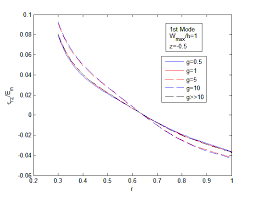The image above represents dimensionless radius.

To compute for the dimensionless radius, two essential parameters are needed and these parameters are external radius (re) and well radius (rw).

The formula for calculating the dimensionless radius:

rD = re / rw

Where;

Let’s solve an example;
Given that the external radius is 18 and the well radius is 16.

This implies that;

re = External Radius = 18
rw = Well Radius = 16

rD = re / rw
rD = 18 / 16
rD = 1.125

Therefore, the dimensionless radius is 1.125.

re = rD x rw

Where;

Let’s solve an example;
Given that the dimensionless radius is 9 and the well radius is 20.

This implies that;

rD = Dimensionless Radius = 9
rw = Well Radius = 20

re = rD x rw
re = 9 x 20
re = 180

Therefore, the external radius is 180.

## How to Calculate and Solve for Gas Formation Volume Factor (FVF) | The Calculator Encyclopedia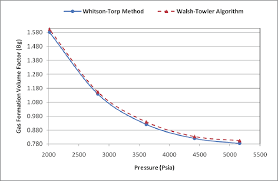The image above represents the gas FVF.

To compute for the gas FVF, three essential parameters are needed and these parameters are Z-Factor (Z), Temperature (T) and Pressure (P).

The formula for calculating the gas FVF:

Bg = 0.00504[ZT / P]

Where;

Bg = Gas FVF
Z = Z-Factor
T = (°R) Temperature
P = Pressure

Let’s solve an example;
Find the Gas FVF when the Z-Factor is 12, temperature is 30 and the pressure is 120.

This implies that;

Z = Z-Factor = 12
T = (°R) Temperature = 30
P = Pressure = 120

Bg = 0.00504[ZT / P]
Bg = 0.00504[12 x 30 / 120]
Bg = 0.00504[360 / 120]
Bg = 0.00504
Bg = 0.01512

Therefore, the gas FVF is 0.01512 bbl / scf.

Calculating for the Z-Factor when the Gas FVF, Temperature and Pressure is Given.

Z = Bg.P / 0.00504T

Where;

Z = Z-Factor
Bg = Gas FVF
T = (°R) Temperature
P = Pressure

Let’s solve an example;
Find the Z-Factor when the Gas FVF is 22, temperature is 16 and the pressure is 80.

This implies that;

Bg = Gas FVF = 22
T = (°R) Temperature = 16
P = Pressure = 80

Z = Bg.P / 0.00504T
Z = 22 x 80 / 0.00504 x 16
Z = 1760 / 0.08064
Z = 21825.39

Therefore, the Z-Factor is 21825.39.

Calculating for the Temperature when the Gas FVF, Z-Factor and the Pressure is Given.

T = Bg.P / 0.00504Z

Where;

T = (°R) Temperature
Z = Z-Factor
Bg = Gas FVF
P = Pressure

Let’s solve an example;
Find the Temperature when the Gas FVF is 44, Z-Factor is 29 and the pressure is 70.

This implies that;

Z = Z-Factor = 29
Bg = Gas FVF = 44
P = Pressure = 70

T = Bg.P / 0.00504Z
T = 44 x 70 / 0.00504 x 29
T = 3080 / 0.14616
T = 21072.79

Therefore, the temperature is 21072.79.

## How to Calculate and Solve for Well Bore Storage Factor, Fluid Density and Well Annulus Cross-Sectional Area in Well Testing | The Calculator Encyclopedia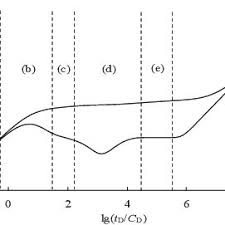The image above represents the well bore storage factor.

To compute for the well bore storage factor, two essential parameters are needed and these parameters are Well Annulus Cross-Sectional Area (Ao) and Fluid Density (ρ).

The formula for calculating the well bore storage factor:

C = 144Ao / 5.615ρ

Where;

C = Well Bore Storage Factor
Ao = Well Annulus Cross-Sectional Area
ρ = Fluid Density

Let’s solve an example;
Find the well bore storage factor when the well annulus cross-sectional area is 54 and fluid density is 42.

This implies that;

Ao = Well Annulus Cross-Sectional Area = 54
ρ = Fluid Density = 42

C = 144Ao / 5.615ρ
C = 144 x 54 / 5.615 x 42
C = 7776/235.83
C = 32.97

Therefore, the well bore storage factor is 32.97.

Calculating the Well Annulus Cross-Sectional Area when the Well bore Storage Factor and the Fluid Density is Given.

Ao = C x 5.615ρ / 144

Where;

Ao = Well Annulus Cross-Sectional Area
C = Well Bore Storage Factor
ρ = Fluid Density

Let’s solve an example;
Find the well annulus cross-sectional area when the well bore storage factor is 34 and fluid density is 24.

This implies that;

C = Well Bore Storage Factor = 34
ρ = Fluid Density = 24

Ao = C x 5.615ρ / 144
Ao = 34 x 5.615 x 24 / 144
Ao = 4581.84 / 144
Ao = 31.81

Therefore, the well annulus cross-sectional area is 31.81.

## How to Calculate and Solve for Crude Oil Gravity, Oil Density and Water Density | The Calculator Encyclopedia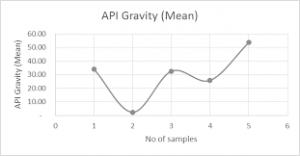The image above represents crude oil gravity.

To compute for the crude oil gravity, two essential parameters are needed and these parameters are oil density (ρo) and water density (ρw).

The formula for calculating the crude oil gravity:

γo = ρo / ρw

Where;

γo = Crude Oil Gravity
ρo = Oil Density
ρw = Water Density

Let’s solve an example;
Find the crude oil gravity when the oil density is 28 and the water density is 46.

This implies that;

ρo = Oil Density = 28
ρw = Water Density = 46

γo = ρo / ρw
γo = 28 / 46
γo = 0.608

Therefore, the crude oil density is 0.608.

Calculating the Oil Density when the Crude Oil Gravity and the Water Density is Given.

ρo = γo x ρw

Where;

ρo = Oil Density
γo = Crude Oil Gravity
ρw = Water Density

Let’s solve an example;
Find the oil density when the crude oil gravity is 30 and the water density is 16.

This implies that;

γo = Crude Oil Gravity = 30
ρw = Water Density = 16

ρo = γo x ρw
ρo = 30 x 16
ρo = 480

Therefore, the oil density is 480.

## How to Calculate and Solve for Total Production Time, Stabilized Well Flow, Cumulative Production before Shut-In | Well Testing | Nickzom Calculator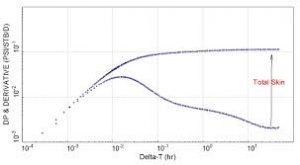The image represents the total production time.

To compute for the total production time, two essential parameters are needed and these parameters are Cumulative Production before Shut-In (Np) and Stabilized Well Flow (Qo).

The formula for calculating the total production time:

tp = 24Np / Qo

Where:

tp = Total Production Time
Np = Cumulative Production before Shut-In
Qo = Stabilized Well Flow

Let’s solve an example;
Find the total production time when the cumulative production before Shut-In is 24 and the stabilized well flow is 43.

This implies that;

Np = Cumulative Production before Shut-In = 24
Qo = Stabilized Well Flow = 43

tp = 24Np / Qo
tp = 24 x 24 / 43
tp = 576 / 43
tp = 13.39

Therefore, the total production time is 13.39 hrs.